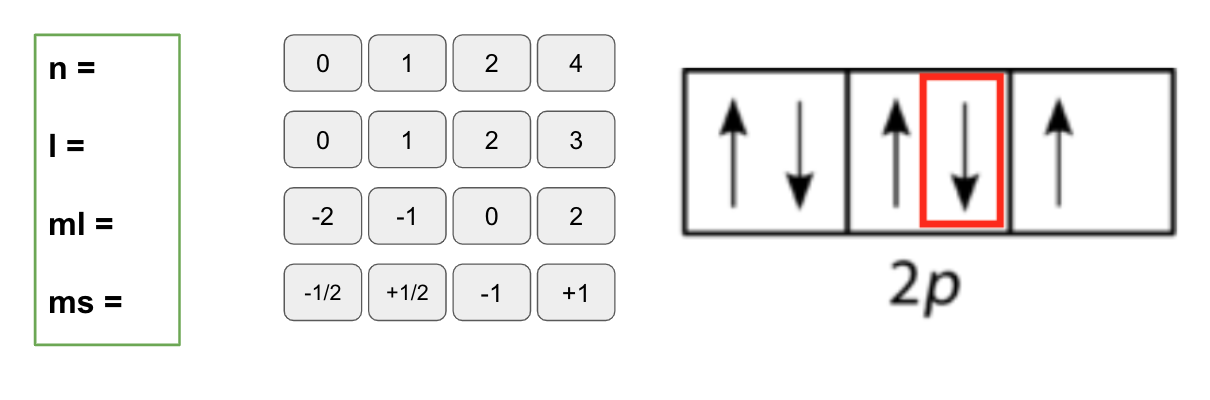# Problem: Find all four quantum numbers (n, l, ml, and ms) for the selected electron below. Select the correct answer for each quantum number.

###### FREE Expert Solution
82% (337 ratings)
###### FREE Expert Solution

For 2p subshell:

2 → n = 2

p → l = 1

82% (337 ratings)###### Problem Details

Find all four quantum numbers (n, l, ml, and ms) for the selected electron below. Select the correct answer for each quantum number.What scientific concept do you need to know in order to solve this problem?

Our tutors have indicated that to solve this problem you will need to apply the The Spin Quantum Number concept. You can view video lessons to learn The Spin Quantum Number. Or if you need more The Spin Quantum Number practice, you can also practice The Spin Quantum Number practice problems.

What is the difficulty of this problem?

Our tutors rated the difficulty ofFind all four quantum numbers (n, l, ml, and ms) for the sel...as medium difficulty.

How long does this problem take to solve?

Our expert Chemistry tutor, Sabrina took 4 minutes and 2 seconds to solve this problem. You can follow their steps in the video explanation above.

What professor is this problem relevant for?

Based on our data, we think this problem is relevant for Professor Keister's class at UB.